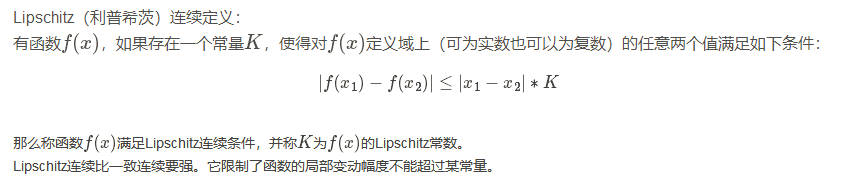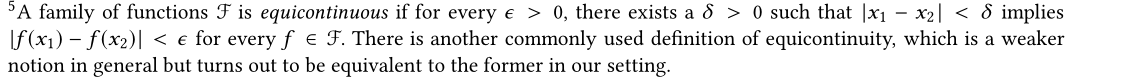• 文章目录一致连续连续与一致连续一致连续的判定一致连续与开区间 一致连续 连续与一致连续 尽管我们可以说函数f(x)f(x)f(x)在区间XXX上连续，但实际上连续的作用目标是“区间上的每一个点”，即 ∀x0∈X,∀ε>0,...
文章目录一致连续连续与一致连续一致连续的判定一致连续与开区间
一致连续
连续与一致连续
尽管我们可以说函数$f(x)$在区间$X$上连续，但实际上连续的作用目标是“区间上的每一个点”，即
$\forall x_0\in X,\forall \varepsilon>0,\exist \delta(\varepsilon,x_0)>0,{\rm s.t.}\forall x\in U(x_0;\delta)\cap X,|f(x)-f(x_0)|<\varepsilon.$
注意到这里$\delta$的选取不止依赖于$\varepsilon$，还依赖于作用的点$x_0$，因此，函数连续依然被视作是点性质，与函数极限类似。
一致连续是一个定义在区间上的概念，不同于连续。也就是说，如果在根据$\varepsilon$寻找$\delta$时只考虑$\varepsilon$的大小，而不考虑选取的具体点$x_0$，即找到一个对任何$x_0$都适用的$\delta(\varepsilon)$，就称$f(x)$在区间$X$上具有一致连续性，即
$\forall \varepsilon>0,\exist \delta(\varepsilon)>0,\forall x_0\in X,{\rm s.t.}\forall x\in U(x_0;\delta)\cap X,|f(x)-f(x_0)|<\varepsilon.$
其等价的定义是：设函数$f(x)$定义在区间$X$上，若对于任意给定的$\varepsilon>0$，存在$\delta>0$，只要$x',x''\in X$满足$|x'-x''|<\delta$，就成立$|f(x')-f(x'')|<\varepsilon$，就称$f(x)$在区间$X$上一致连续。
可以看出：$f(x)$在$X$上一致连续$\Rightarrow f(x)$在$X$上连续，但反之不一定成立。这是因为$\forall x_0\in X$，可以取$\delta(x_0,\varepsilon)=\delta(\varepsilon)$，就得到$f(x)$在点$x_0$的连续性，进而得到$f(x)$在区间$X$上的连续性。而反之，可以找出一些在开区间上的例子，说明$f(x)$在开区间上连续但不一致连续，如
$f(x)=\frac 1x,\quad x\in (0,1].$
要使得$|f(x)-f(x_0)|<\varepsilon$，就有
$\left|\frac 1x-\frac1{x_0}\right|<\varepsilon,\quad \frac 1{x_0}-\varepsilon<\frac 1x<\frac 1{x_0}+\varepsilon\\ \Downarrow\\ \frac{x_0}{1+\varepsilon x_0}
得到$\delta(x_0,\varepsilon)=\frac{x_0^2\varepsilon}{1+x_0\varepsilon}$，这是$\delta$的精确解，可以看出，$x_0\to 0$时$\delta(x_0,\varepsilon)\to0$，所以不存在一个对所有$x_0$都适用的$\delta(\varepsilon)$，故$f(x)$在$(0,1]$上不是一致连续的。
但对于闭区间，有Cantor定理保证，$f(x)$在$X$上连续$\Leftrightarrow f(x)$在$X$上一致连续。
而对于有限开区间$(a,b)$，只要$f(a^+),f(b^-)$存在且有限，那么就可以将其延拓成闭区间，因此一样能由连续性推出一致连续性。
一致连续的判定
刚才我们得出了一种计算$\delta(x_0,\varepsilon)$的精确解，来判断是否一致连续的方法，但精确解的计算是比较困难的，在绝大多数时候会对$\delta$进行放缩。有一种更简便的方法来判断非一致连续性。
命题：设$f(x)$在区间$X$上定义，则$f(x)$在$X$上一致连续的充要条件是，对任何$X$上的点列$\{x_0'\},\{x_0''\}$，只要满足$\lim\limits_{n\to \infty}(x_n'-x_n'')=0$，就成立$\lim\limits_{n\to \infty}[f(x')-f(x'')]=0$。
证明：先证明必要性。
$f(x)$在区间$X$上一致连续，即$\forall \varepsilon>0,\exists \delta(\varepsilon)>0$，只要$|x'-x''|<\delta$，就有$|f(x')-f(x'')|<\varepsilon$。现既然$\lim\limits_{n\to \infty}(x_n'-x_n'')=0$，则对于任何$\delta(\varepsilon)>0$，必定存在一个$N(\varepsilon)$当$n>N$时，$|x'_n-x_n''|<\delta(\varepsilon)$，于是$|f(x_n')-f(x_n'')|<\varepsilon$。
再证明充分性，采用反证法。
如果对于不一致连续的函数$f(x)$，一定存在$X$上的点列$\{x_n'\},\{x_n''\}$，虽然$x_n'-x_n''\to 0$，但是$f(x_n')-f(x_n'')\nrightarrow 0$，就可以证明原命题的成立。因此，我们考虑一个不一致连续的函数$f(x)$，按照不一致连续的定义，有
$\exists \varepsilon_0>0,\forall \delta>0,\exist x',x''\in X,|x'-x''|<\delta,|f(x')-f(x'')|>\varepsilon_0.$
取一列$\{\delta_n\}\to 0$，不妨取$\delta_n=\dfrac 1n$，则有
$|x_n'-x_n''|<\delta_n\to 0,\quad |f(x_n')-f(x_n'')|>\varepsilon_0.$
这就得到了点列满足条件，因此不一致连续的函数一定存在距离无限接近但函数值不接近的点列，也就是只要所有无限接近的点列函数值都无限接近，那么一定是一致连续。
一致连续与开区间
刚才我们说过，对于有限开区间$(a,b)$，只要其端点处存在单侧极限，就能从连续推出一致连续。然而，这个命题反过来也是成立的，即对于开区间$(a,b)$上的一致连续函数$f(x)$，可以推出$f(a^+),f(b^-)$存在且有限。
因为$f(x)$在开区间$(a,b)$上一致连续，所以$\forall \varepsilon>0,\exist \delta$，当$|x'-x''|<\delta$时$|f(x')-f(x'')|<\varepsilon$。在区间$(a,b)$上任意选取数列$x_n\in (a,b)$且$\lim\limits_{n\to \infty}x_n=a$，这里$\{x_n\}$是基本列，所以对于上述$\delta$，
$\exist N,\forall n,m>N:|x_n-x_m|<\delta \Rightarrow |f(x_n)-f(x_m)|<\varepsilon.$
这就说明$f(x_n)$也是基本数列，存在极限。由Heine定理，$\lim\limits_{x\to a^+}f(x)$存在且有限，记作$f(a^+)$即可 。同理可以证明$\lim\limits_{x\to b^-}f(x)$存在且有限，记作$f(b^-)$。
但对于无限开区间，不能得到这个结果，因为Cauchy收敛准则的收敛是指对有限数的收敛，对无穷的收敛是不适用的，如取$\{x_n\},x_n=-n$，就不存在这样的$N$使得$|x_n-x_m|<\varepsilon$。
可以取一个在无穷区间上一致连续，但不存在无穷极限的，如$f(x)=\sin x$在$\R$上一致连续，但不存在极限。但如果单侧无限区间$[a,+\infty)$或$(-\infty,b]$上存在极限$A=\lim\limits_{x\to +\infty}f(x)$或$B=\lim\limits_{x\to -\infty}f(x)$，那么$f(x)$在这样的无限区间上是一致连续的。


展开全文• 一致连续的直白意义是： 若函数 fff 一致连续，对于任意两点 xxx 与 yyy，只要 xxx 与 yyy 充分接近，f(x)f(x)f(x) 与 f(y)f(y)f(y) 也能够充分接近。 邻域定义： 对于任意实数 ϵ&gt;0\epsilon&gt;0ϵ>0...
一致连续的直白意义是： 若函数 $f$ 一致连续，对于任意两点 $x$ 与 $y$，只要 $x$ 与 $y$ 充分接近，$f(x)$ 与 $f(y)$ 也能够充分接近。
邻域定义：
对于任意实数 $\epsilon>0$，总存在实数 $\delta>0$，只要 $\|x-y\|<\delta$，都有 $\|f(x)-f(y)\|<\epsilon$.
海涅-康托尔定理表面：若一个函数在闭区间连续，则该函数也是一致连续的。


展开全文实函数
• 连续、一致连续、一致收敛等度连续是函数或函数列非常重要的性质。针对收敛的函数列，探讨了一致连续、一致收敛等度连续两两之间的关系，并在有界区间上给出了一致连续、一致收敛等度连续的等价关系。
• 1.函数连续 定义：设函数y=f(x)y=f(x)y=f(x)在点x0x_0x0​的某一个领域内有定义，如果 lim⁡Δx→0Δy=lim⁡Δx→0[f(x0+Δx)−f(x0)]=0, \lim_{\Delta x \rightarrow 0}\Delta y = \lim_{\Delta x \rightarrow 0} \...
1.函数连续
定义：设函数$y=f(x)$在点$x_0$的某一个领域内有定义，如果
$\lim_{\Delta x \rightarrow 0}\Delta y = \lim_{\Delta x \rightarrow 0} \left[ f\left(x_0+\Delta x\right) -f\left(x_0\right) \right] = 0,$
那么就称函数$y=f\left( x \right)$在点$x_0$连续。
2.区间连续
定义：如果在区间上每一点都连续，就称函数在该区间上连续，如果区间包括端点，那么在右端点连续是指左连续，在左端点连续是指右连续。
3.一致连续
定义：设函数$f\left(x \right)$在区间$I$上有定义，如果对于任意给定的正数$\varepsilon$，总存在正数$\delta$，使得对于区间$I$上的任意两点$x_1$，$x_2$ ，当$\left|x_1-x_2\right| < \delta$时，有
$\left|f(x_1)- f(x_2)\right|<\varepsilon$那么称函数$f(x)$在区间$I$上一直连续。
4.绝对连续
设$f(x)$是$[a,b]$上的函数，若对任意$\varepsilon>0$，存在$\delta>0$使得对于$[a,b]$中的任意一组分点：$a_{1}只要$\sum_{i=1}^{n}(b_i-a_i)<\delta$，便有$\sum_{i=1}^{n} \mid f\left(b_{i}\right)-f\left(a_{i}\right)|<\varepsilon，$则称$f(x)$是$[a,b]$上的绝对连续函数，或称$f(x)$在$[a,b]$上绝对连续。
5.Lipschitz连续
对于函数$f(x)$，如果存在一个常量$K$，使得对$f(x)$定义域上（可为实数也可以为复数）的任意两个值满足如下条件：
$\left|f(x_1)-f(x_2)\right| \leq K*\left|x_1-x_2\right|$那么称函数$f(x)$满足Lipschitz连续条件，并称$K$为$f(x)$的Lipschitz常数。


展开全文数学
• .1. 2.
.1.2.展开全文• 函数的一致连续性是数学分析中最重要,且高度抽象的概念之一,在数学分析相关专业课的后继学习与研究中起着十分重要的作用。为了帮助学生深刻理解一致连续性的概念,首先,从连续的概念出发,形象直观地阐述一致连续性...
• 这就是我们要介绍的一致连续函数(uniformly continuous function)，精确的定义如下。定义3\textbf{定义3} 令f:A→Rm,B⊂Af:A\to R^m,B\subset A，我们说ff 在集合BB上一致连续，如果对每个ε>0\varepsilon>0，存在δ...
• 基础比较差，常常搞不清楚几个概念，自己写一写有助于记忆理解，水平有限，疏漏之处欢迎大家批评指正~ 1 连续 个人理解，连续是个局部的概念，一般说函数在某一点x0处，满足那样一个性质，就说这个函数在x0连续。 ...
• 针对函数一致连续证明问题,给出了证明方法的流程图,该流程图对函数一致连续性证明给出了一个清晰的思路,通过例题解释流程图使用方法。事实表明该流程图对函数一致连续证明是有效的。
• 函数一致连续性的定义 关于函数一致连续性，准确的定义如下： 设函数 在区间 上有定义，如果对于任意给定的正数 ，总存在着正数 ，使得对于区间上的任意两点，，当时，就有，那么称函数 在区间 上是一致连续的。 ...
• 引人了一致连续偏序集的基的概念,给出了其一些等价刻画,讨论了一致连续偏序集的权与相应一致Scott拓扑空间的权之间的关系,并且进一步讨论其与相应的一致Lawson拓扑空间的权之间的关系最后给出了在一致连续偏序集中,...
•  大家知道，函数f在闭区间[a，b]上的一致连续性是指：对任意ε>0，必存在一个δ.>0，只要│x'– x''│，则必有│f(x')-f(x'')│< ε。  康托尔定理是断言：函数f在闭区间[a，b]上处处连续，则必一致连续
• 设$[a,b]$是$\mathbf{R}$上的闭区间,且$f(x)$是$[a,b]$上的连续函数,则$f(x)$在$[a,b]$上一致连续. 证明:反证法.假设$f(x)$在$[a,b]$上不是一致连续的,则必定存在这么两个序列,其中$$a_1,a_2,\cdots,a_n,\cdots$$...
• 设 $(X,d_X)$ $(Y,d_Y)$ 都是度量空间,假定 $(X,d_X)$ 是紧致的,如果 $f:X\to Y$ 是函数,那么 $f$ 是连续的当且仅当 $f$ 是一致连续的. 证明:当 $f$ 是一致连续时,$f$ 显然是连续的.我们主要证明 $f$ 连续时一致...
• 3. 一致连续与连续的关系：若函数在开区间内一致连续，则其在该区间内连续 4. 一致连续的几何解释（例：正弦函数的一致连续性） 5. 康托尔定理、聚点原理、函数在开区间内一致连续的充要条件 ...
• 7 二阶多智体系统一致性7.1 连续时间多智能体系统一致性7.1.1 协议分析7.1.2 仿真实验7.2 带有领航者多智能体系统一致性7.2.1 协议分析7.2.2 仿真实验7.3 编队多智能体系统一致性7.3.1 协议分析7.3.2 仿真实验7.4 ...
• 6.1 连续时间多智能体系统一致性 6.1.1 协议分析 6.1.2 仿真实验 6.2 离散时间多智能体系统一致性 6.2.1 协议分析 6.2.2 仿真实验 6.3 连续时间含时滞系统一致性 6.3.1 协议分析 6.3.2 仿真实验 6.4 切换拓扑系统...
• 设$f:\mathbf{R}\to \mathbf{R}$是可微函数，并且$f'$是有界的，证明$f$是一致连续的. 证明:设 \begin{align*} a_1,a_2,\cdots,a_n,\cdots \end{align*}是$\mathbf{R}$上的任意一个数列.设 \begin{align*} b_1,b_...
• 7 二阶多智体系统一致性7.1 连续时间多智能体系统一致性7.1.1 协议分析7.1.2 仿真实验7.2 带有领航者多智能体系统一致性7.2.1 协议分析7.2.2 仿真实验7.3 编队多智能体系统一致性7.3.1 协议分析7.3.2 仿真实验7.4 ...
• 连续子序列的最大 前言 分治算法的核心思想是将一个规模很大的问题化简为n个规模较小的问题，这些子问题虽然独立而不同，但是问题的本质是一致的，从而达到分而治之的目的。 首先通过“分”将问题分解为n个子......